Asynchronous + Flexible Enrollment = Work At Your Own Best Successful Pace

# Precalculus Online Course with Trigonometry for Academic Credit

Precalculus - DMAT 135 - Computational Precalculus with Trigonometry is a catch-all phrase for all of the algebra and trigonometry usually studied in high school.

Course: DMAT 135 - Computational Precalculus with Trigonometry 
Cost: $1732 Tuition +$70 Semester Fee + \$115 Software/E-textbook

Delivery: Fully Online, Asynchronous, Self-Paced

The topics of any standard Precalculus course will include:
• Solving Equations (both easy and more difficult)
• Linear Equations
• Polynomials
• Factoring Polynomials
• Rational Polynomials
• Functions
• Exponential and Logarithmic Functions
• Trigonometry Concepts
• Trigonometric Functions
• Systems of Equations - Linear and More Complicated Equations
• Complex Numbers
• Equations and Inequalities
• Conic Sections

Completion of the Distance Calculus @ Roger Williams University DMAT 135 - Computational Precalculus with Trigonometry course earns 4 academic credit semester hours, earning an official academic transcript from Roger Williams University, in Providence, Rhode Island, USA, which is regionally accredited by the New England Commission of Higher Education (NECHE), facilitating transfer of credits nationwide to other colleges and universities.

## Course Matrix: DMAT 135 - Computational Precalculus with Trigonometry

Prerequisite
THIS LEVEL
Next Courses
 → Legacy Course MATH 136 = DMAT 136:Precalculus Mathematics[4 credits]
 → DMAT 253:STEM Calculus I[4 credits] Honors DMAT 254:Honors STEM Calculus I[5 credits] Honors DMAT 255:Honors Calculus I+II for Data Science[5 credits]

## Online Precalculus Course for College Credit Introductory Video

Distance Calculus @ Roger Williams University - Math 136 - Precalculus is a self-paced, asynchronous, live-lecture-free, high-tech, empirical, investigation-driven approach to the traditional topics of high-school (and lower college) Precalculus.

## The Distance Calculus Approach To Precalculus

Our online Precalculus course Math 136 from Distance Calculus @ Roger Williams University has the following educational assumptions and goals:

Distance Calculus is intended for adult students (although we do have advanced High School students in the upper courses like Multivariable Calculus, Differential Equations, Linear Algebra, and Probability Theory), and our Precalculus course is designed for adult learners - especially those looking to continue into our Engineering Calculus sequence.
• Rote Learning Should Be Left in High School
All Distance Calculus students have already gone through high school. Repeating such a high school "rote skills" Precalculus course seems unlikely to produce success to adult learners
• Computer Algebra and Graphing Throughout The Curriculum
As all Distance Calculus courses are based upon curricula written in LiveMath and/or Mathematica - both powerful computer algebra and graphing systems, we extend these tools in the Precalculus course which change the nature of the curriculum, far more than just using a simple graphing calculator as an ancillary tool to a traditional "rote" curriculum
• Empirical Learning
Instead of a "proofs-based" Precalculus course, we use the powerful computer algebra and graphing platform LiveMath to conduct graphical, numerical, and algebraic experiments - like you would in a biology or chemistry or physics laboratory - to empirically investigate the topics of traditional high school precalculus. "Rote" learning is replaced with investigating the topics much more thoroughly using modern technical tools at a much deeper level, because the "Do these 1-99 odd factoring problems" is now replaced with "Use Factor Command" - and then we ask when we can use factoring, what it does for us, and what do we do when we cannot factor!
• Asynchronous Studying
This means that the course happens on your schedule, not at fixed times. You may work as intensely as you wish, take a break for a week or two, and then resume, all on your needs.
• Mastery Learning
The student will learn the current module of material thoroughly before moving on to the next topic. This eliminates the "slippery slope" problem of not mastering a previous topic when faced with a new topic.
• Synchronous Communications
The majority of communcation between the student and the instructors will occur via Chat, so that problems, questions, issues, that come up are solved in real time.
• ChalkTalk Video
Video presentations of problems solved manually, "by hand", providing the basis of the classical study of precalculus topics - we have a balance of 80% Computer Algebra and 20% By Hand Manual Computations split of the problems. There are core problems that all students who complete a Precalculus course should be able to do by hand, which is reinforced by the Computer Algebra assignments.

## Examples of Distance Calculus Unique Approach in Precalculus

Many prospective students will have completed Algebra at some level in high school, perhaps many years ago, and they are looking for a comprehensive course on Precalculus before they start the Engineering Calculus I sequence. Below we list a few topics that demonstrate the enhanced curriculum of our Precalculus course, which studies the same topics as high school Algebra and Trigonometry, but we are able to go to much deeper levels of investigation because of the usage of the computer algebra platform LiveMath.

• Factoring A Polynomial By Graphing Analysis
Factoring is now made rather trivial using LiveMath's Factor command:You would never attempt to factor such a polynomial in high school algebra class by hand! After we make the standard connection to the x-axis crossings/touches for this polynomial function graphed: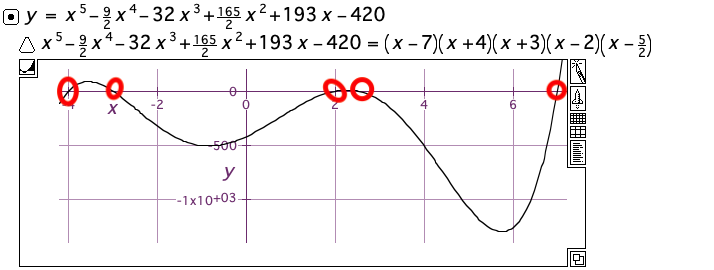We then ask the question in reverse:
Suppose you are given the graph of a function whose algebraic formula you do not know - can you reconstruct the algebraic formula (assuming it is a polynomial function) from just the graph?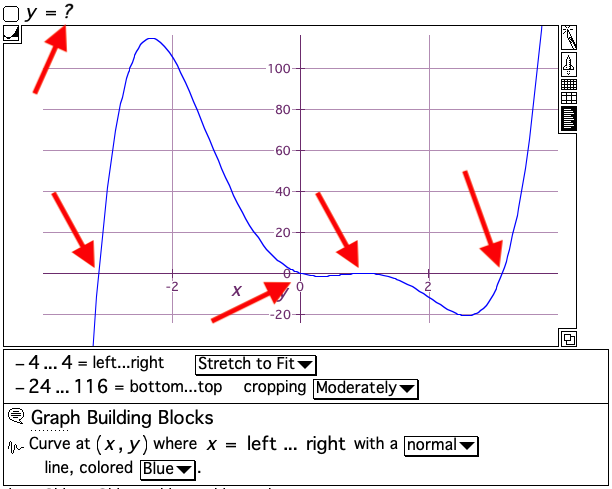• How Complex Roots Affect A Polynomial Function Graph
Here's what looks to be a degree 4 polynomial function - can we find its algebraic formula?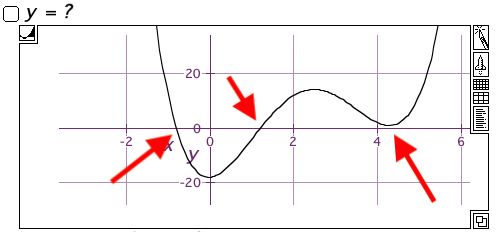Only problem is: there are only 2 real x-axis crossings! That function wiggles, but not far enough down to actually cross the x-axis! Turns out this wiggle is the affect of complex roots at work. But we can still analyze this graphically by vertically translating the graph down to force that wiggle to cross the x-axis (and cross at nice roots, too!):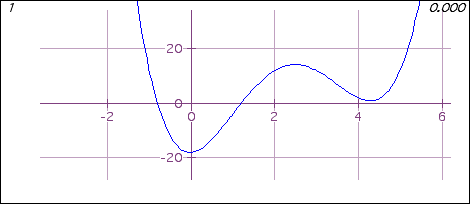From that translation we can get 4 real roots, create the factorization, and then ... don't forget ... reverse the vertical translation back up, and voila!• Conic Sections - Parabolas in 3D Animations
Remember parabolas, hyperbolas, ellipses in high school algebra? Well, they never looked this cool in 3D animations on those little TI-83 calculators!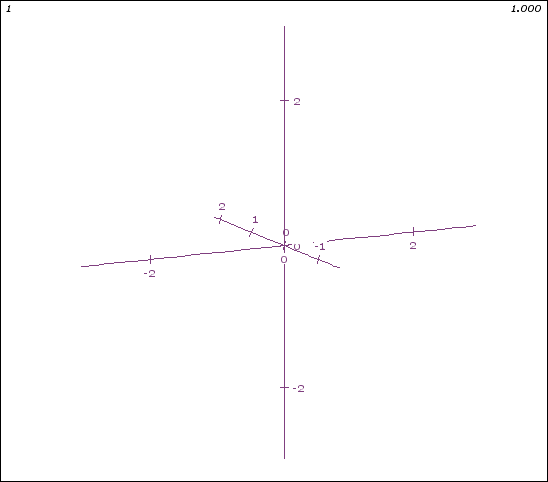In our Precalculus course, the equations and analytical geometry study of parabolas, hyperbolas, and ellipses takes on a 3D feel, where we study much harder problems: how do we convert these slicing equations into standard form, and rotate everything "just so" to get the standard equations to pop out?

## Other Online Precalculus Courses vs. Distance Calculus

The Distance Calculus Precalculus online course was created as a support course for our Calculus I - Engineering Calculus sequence. Many students who need to complete Calculus I or higher in the engineering sequence are often not prepared for starting Calculus I - especially the considerably-different Calculus I online course offered via Distance Calculus.

There are many Precalculus online courses available around the internet. In almost all cases, these other Precalculus online courses are just a re-hash of the high school algebra II and trigonometry experience - more of the same - endless hand-calculation problem sets ("1-99 odd"), perhaps with usage of a graphing calculator (high school Precalculus courses now routinely include the Texas Instrument TI-83 or similar graphing calculators), and probably with a multiple choice engine where your instructor becomes an automated machine checking answers instead of a human being.

These other online Precalculus online courses all share the same process: a paper or e-paper textbook is provided to the student, and long problem sets of factoring polynomials, applying the quadratic formula, interpreting word problems into solvable equations, etc.

The Educational term for these courses is: learning by rote - doing a hand-technique over and over again, until the technical process is imprinted in your mind. Many students who go through these types of precalculus courses are so trained that when they see an equation like the following:

x2 + 5x - 8 = 0
They immediately say "Oh, I know the quadratic formula:"

$$\frac {-b\pm\sqrt{b^2 - 4ac}}{2a}$$

But often these students are completely stumped what to do if the equation instead looks like this:

x3 + 5x + 8 = e2x
There often is a disconnect between generally solving equations for (numerical) solutions, and applying (historical) formulas that work only in special cases (purely quadratic equations).

Many students are very successful at "rote" Precalculus courses. As almost all of the other Precalculus online courses on the internet are exactly these "rote" courses, if you are looking for such a "plug-and-chug" Precalculus online course, you have many options, and Distance Calculus is not for you.

If, on the other hand, diving back into another high-school-like Precalculus "rote" course does not sound appealing to you, then read on about our Distance Calculus Precalculus online course.

## Roger Williams University Course Catalog Listing: DMAT 135 - Computational Precalculus with Trigonometry

Course: DMAT 135

Course Title: Computational Precalculus with Trigonometry

Transcript Course Title (30 Characters Max:): Comp Precalc with Trigonometry

Course Description: A single course on the study of linear, quadratic, polynomial, rational, exponential, logarithmic, and trigonometric functions via algebraic, numerical, graphical, and narrative viewpoints, preparing the student for the Calculus sequence. [4 Semester Credits]

Prerequisite: Successful completion of 3 years high school mathematics (C- or higher) or instructor consent.

E-Textbook: “The Primitives of Precalculus” by Robert R. Curtis, Ph.D.

Software: LiveMath

### DMAT 135 - Computational Precalculus with Trigonometry - Learning Outcomes

• 1. To identify, manipulate, and understand the algebraic, numerical, and graphical fundamentals of linear functions, including slope, intercepts, linear growth, and intersections of linear plots.
• 2. To identify, manipulate, and understand the algebraic, numerical, and graphical fundamentals of quadratic functions, including standard form, vertices, factoring, the quadratic formula, and complex numbers.
• 3. To identify, manipulate, and understand the algebraic, numerical, and graphical fundamentals of polynomial functions, including factoring, roots, polynomial growth, inverse functions, and the Fundamental Theorem of Algebra
• 4. To identify, manipulate, and understand the algebraic, numerical, and graphical fundamentals of rational polynomial functions, including asymptotes, common roots, solving equations.
• 5. To identify, manipulate, and understand the algebraic, numerical, and graphical fundamentals of inequalities, including solving inequalities, ranges, systems of inequalities.
• 6. To identify, manipulate, and understand the algebraic, numerical, and graphical fundamentals of exponential and logarithmic functions, including bases, exponential and logarithmic growth, solving equations involving exponentials and logarithms, and inverse function relations.
• 7. To identify, manipulate, and understand the algebraic, numerical, and graphical fundamentals of trigonometric functions, including trigonometric ratio definitions, the six trigonometric functions, trigonometric identities, solving trigonometric equations, and applications of trigonometry.
• 8. To identify, manipulate, and understand the algebraic, numerical, and graphical fundamentals of conic sections, including intersections of planes and cones, the classical conic section types, and transformation of coordinates.

### DMAT 135 - Computational Precalculus with Trigonometry - Syllabus of Topics

1.	Getting Started
1.1.	Email and Chat
1.3.	Required Computer Hardware
1.4.	Software Fundamentals

2.	The Big Picture
2.1.	Solving (easy) equations in 1 variable.
2.2.	What if you can't solve for x?
2.3.	Finding solutions numerically
2.4.	Finding solutions graphically
2.5.	Solving equations of more than 1 variable

3.	Functions
3.1.	Function notation
3.2.	Data sets
3.3.	Graphing functions
3.4.	Data sets and smooth curves
3.5.	Domain and Range
3.6.	Algebraic combinations of functions

4.	Linear Functions
4.1.	Algebraic definition
4.2.	Slope
4.3.	Graphing linear functions by hand
4.4.	Properties of linear functions
4.5.	Linear data sets

5.1.	Algebraic definition
5.2.	Graphing and Properties of Quadratic Functions
5.5.	Solving quadratic equations numerically and graphically
5.6.	Complex Numbers

6.	Power and Polynomial Functions
6.1.	Algebraic definition
6.2.	Graphing and Properties of Polynomial Functions
6.3.	Solving polynomial equations algebraically: factoring
6.4.	Solving polynomial equations numerically and graphically
6.5.	Fundamental Theorem of Algebra

7.	Rational Polynomial Functions
7.1.	Algebraic definition
7.2.	Graphing and Properties of Rational Polynomial Functions
7.3.	Solving rational polynomial equations algebraically: factoring
7.4.	Solving rational polynomial equations numerically and graphically

8.	Inequalities, Systems of Equations
8.1.	Inequalities of 1 variable
8.2.	Inequalities of 2 variables
8.3.	System of Equations in 2 variables

9.	Introduction to Matrices
9.1.	Inverses
9.2.	Determinants
9.3.	Cramer's Rule

10.	Exponential Functions
10.1.	Algebraic definition
10.2.	Graphing and Properties of Exponential Functions
10.3.	Solving exponential equations numerically and graphically
10.4.	Exponential Growth and Applications
10.5.	Data sets and exponential functions

11.	Logarithmic Functions
11.1.	Inverse Functions
11.2.	Algebraic Definition
11.3.	Graphing and Properties of Logarithmic Functions
11.4.	Solving exponential and logarithmic equations algebraically
11.5.	Solving logarithmic equations numerically and graphically
11.6.	Logarithmic Growth and Applications
11.7.	Data sets and logarithmic functions

12.	Trigonometry
12.1.	Geometry of Right Triangles
12.2.	The Unit Circle
12.3.	The (Circular) Trigonometric Functions
12.4.	Graphing and properties of trigonometric functions: frequency, amplitude, shifting
12.6.	Trigonometric identities
12.7.	Inverse Trigonometric functions
12.8.	Solving Trigonometric equations algebraically
12.9.	Solving Trigonometric equations graphically and numerically
12.10.	Applications of trigonometry
12.11.	Laws of sines and cosines
12.12.	DeMoivre's Theorem

13.	Analytic Geometry
13.1.	The Cone
13.2.	Parabolae, Hyperbolae, Ellipsi
13.3.	Polar Coordinates
13.4.	Parametric Equations and Graphs

14.	Sequences and Series
14.1.	Sequences
14.2.	Summation
14.3.	Arithmetic and Geometric Sequences and Series

## Legacy Distance Calculus Course:DMAT 136 - Precalculus Mathematics

In 2023, Distance Calculus introduced a new catalog of courses. The connection between the old courses and the new courses are given here:

New DMAT 135 = OLD DMAT 136 = OLD MATH 136

Legacy Course Description: The focus of this course is on functions, which are of central importance in Calculus. Topics include definitions, properties, and applications of algebraic, inverse, exponential, logarithmic and trigonometric functions. (4 credits)

### Precalculus Online Course Example Learning Movies

One of the ongoing themes of the Precalculus curriculum is bridging between the classical approach to Precalculus with pen/paper - or chalk on a chalkboard - and using the modern LiveMath computer algebra and graphing platform. Often the video assignments come in pairs, with the same core topic explained in both ChalkTalk format and also in LiveMath format.

## Student Work Assignments

Students will complete a variety of types of homework assignments, including:

• Written Solution Sets
Students will complete written solution sets, take a photo with your phone, and turn them in on-line for grading. These problem sets will be from the classical side of the precalculus curriculum - the "math you need to be able to do by hand."

• Computer Algebra Notebook Solution Sets
Students will complete notebook questions in using LiveMath software dealing with more modern types of questions in Precalculus requiring technological approaches.

• Video Solution Sets
Students will complete some Video Solutions of various problems, and turn these videos in on-line for grading, expanding upon both types of solution sets with more of a "Now You Explain It".

Similar to our Distance Calculus course structure, this course is based upon heavy amounts of communication between the student and the instructional team. All assignments are based upon the mastery learning model, and turned in recursively back-and-forth between student and instructor until 100% mastery is achieved.

This is an excellent course for the computer novice. Even if checking your email is a not a "natural" activity for you, the Distance Precalculus course is an excellent way to simultaneously prepare for Calculus, and conquer whatever computer "phobia" fears you may have.

## Distance Calculus - Student ReviewsDate Posted: Apr 6, 2020
Review by: Paul Simmons
Courses Completed: Multivariable Calculus, Differential Equations
Review: I took Multivariable and Diff Eq during the summer. The DiffEq course was awesome - very useful for my physics and engineering course. I was unsure about Mathematica at first, but I got the hang of it quickly. Thank you Distance Calculus!
Transferred Credits to: University of OregonDate Posted: Sep 20, 2020
Review by: Genevieve P.
Courses Completed: Applied Calculus
Review: I found out from my grad school after being accepted that I needed a Calculus course before starting their MBA program. I had less than 6 weeks to do it (and as a non-STEM undergrad no less). The video lectures were informative, the pre-calc refresher was great to get re-conditioned, and the asynchronous format worked so well as I did this at night/weekends after work. I completed it in 4 weeks. Professor Curtis was extremely responsive, graded assignments quickly, and a supportive guide providing constructive feedback to me to excel at the assignments. I highly recommend this course for those who need a pre-req in a hurry or like learning on their own schedule. Thanks, Distance Calculus and Professor Curtis!
Transferred Credits to: Massachusetts Institute of Technology (MIT)Date Posted: Jan 19, 2020
Review by: Dan P.
Courses Completed: Calculus I, Calculus II
Review: I found the courses to be informative, enjoyable, and most importantly, effective in helping me learn the concepts of calculus. My math skills were always very weak, and I had a great deal of difficulty passing my undergrad math courses. The pace of a traditional classroom setting was just too quick for the concepts to really sink in. With Distance Calculus, I had courses that were taught with the full rigor of an on-campus class, but where I could take my time and really learn the material...all while having access to top-tier instructional help for real math professors and assistants. DC gave me the tools and the confidence I needed, so after successfully passing my DC courses, I moved on and completed a master's degree in CS.

No, not really. The student is expected to have "High School Algebra" but nearly all U.S. college/university students have taken High School Algebra, probably Algebra II, as that is a universal requirement for college/university. We do start at the very beginning, and assume that even though you have taken High School Algebra, you may not remember much of it.

Yes. Precalculus is often called "Precalculus With Trigonometry" to remind everyone of the trigonometry component. Essentially Precalculus is, as the name suggests, the course you take before Calculus I.

No. Precalculus is a prerequisite for the higher Calculus I STEM sequence; not for the lower Applied Calculus, single-semester course on calculus.

Well not from Distance Calculus! Our Precalculus course is quite progressive, exploring the topics of Precalculus with a laboratory-minded approach, using graphical, algebraic, and numerical tools to have a refreshing investigation (or re-investigation) of the topics of Precalculus, including Trigonometry.

Yes, All Distance Calculus courses are offered through Roger Williams University in Providence, Rhode Island, USA, which is regionally accredited (the highest accreditation) through New England Commission of Higher Education (NECHE).

Course Level DMAT #
 Course Title Credits
Freshman
Core
253 Calculus I 4
263 Calculus II 4
Freshman
HONORS
254 HONORS Calculus I 5
264 HONORS Calculus II 5
255 HONORS Calculus I+II For Data Science 5
Applied
Calculus